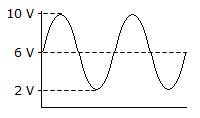# Electronics - Alternating Current and Voltage - Discussion

### Discussion :: Alternating Current and Voltage - General Questions (Q.No.3)

3.What is the peak-to-peak voltage of the waveform in the given circuit?

 [A]. 2 V [B]. 4 V [C]. 6 V [D]. 8 V

Answer: Option D

Explanation:

No answer description available for this question.

 Rajeevkumar said: (Feb 6, 2011) Sir, I want to know the reason to that question please give reply me.

 Randeep Singh said: (Jul 20, 2011) I want to get the answer of this question please explain.

 Sundar said: (Jul 20, 2011) @All In the given Sine wave, Highest Peak voltage value = 10v. Lowest Peak voltage value = 2v. Therefore, Peak-to-peak = 10 - 2 = 8 volts. Another values: Highest Peak voltage value = 10v. Lowest Peak voltage value = -5v. Therefore, Peak-to-peak = 10 - (-5) = 15 volts. Highest Peak voltage value = -10v. Lowest Peak voltage value = -2v. Therefore, Peak-to-peak = (-10) - (-2) = -10 + 2 = -8 = 8 volts. Hope you understand. Have a nice day!

 Ali said: (Dec 16, 2011) What is choke and describe its function?

 Aoun said: (Jul 2, 2013) The choke works in conjunction with a starter. When you turn on the light, voltage is applied to the choke, and when the switch opens after a few seconds, it creates a high voltage across the contacts at each end of the tube. This starts the ionization of the gas, which glows. Once ionized, it continues producing light even when the voltage applied is only 120 VAC, the power line voltage.

 Nagendra Bc said: (Sep 23, 2014) What is peak-to-peak voltage?

 Prince Tetteh said: (Mar 13, 2015) I guess from my own understanding, peak-to-peak voltage is the difference between the maximum voltage level and the minimum voltage level. From the question, the maximum voltage level is 10 V and the minimum voltage level is 2 V. Hence the peak-to-peak voltage is 10-2=8 V.

 Ashish said: (Oct 26, 2015) If both voltages are equal then what will be the answer?

 Arv said: (Jul 14, 2016) Peak to peak will be Zero.

 Obaid said: (Oct 18, 2016) What is the peak to peak value for 120 V ac? Can you please explain in detail?

 Dorah said: (Dec 18, 2016) The maximum peak value ie 10v minus least peak value ie 2v. So, the answer is 8v.

 Power Serge said: (Feb 5, 2017) The zero line is 6V. From 6V to 10V is 4V difference. From 6V to 2V is 4V difference. So, if we add 2 differences, 4+4=8V.

 Ravi said: (Sep 23, 2018) What is a starter?

 Asif said: (Sep 24, 2018) What is the rms value of a given graph?

 Sanjeev said: (Feb 28, 2019) Peak-to-peak (pk-pk) is the difference between the maximum positive and the maximum negative amplitudes of a waveform, as shown below. For an AC sine wave with no DC component, the peak-to-peak amplitude is equal to approximately 2. 828 times the root-mean-square amplitude.

 Hari said: (Mar 3, 2019) Thank you @Sundhar.

#### Post your comments here:

Name *:

Email   : (optional)

» Your comments will be displayed only after manual approval.

#### Current Affairs 2021

Interview Questions and Answers